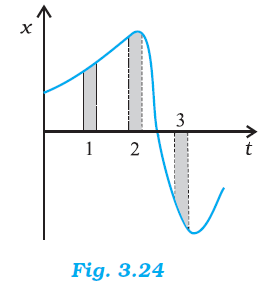## Pages

### Motion in a straight line NCERT Solutions Class 11 Physics - Solved Exercise Question 3.21

Question 3.21

Figure 3.24 gives the x-t plot of a particle in one-dimensional motion. Three different equal intervals of time are shown. In which interval is the average speed greatest, and in which is it the least ? Give the sign of average velocity for each interval.Solution:

Interval 3 (Greatest), Interval 2 (Least)
Positive (Intervals 1 & 2), Negative (Interval 3)
The average speed of a particle shown in the x-t graph is obtained from the slope of the graph in a particular interval of time.

It is clear from the graph that the slope is maximum and minimum restively in intervals 3 and 2 respectively. Therefore, the average speed of the particle is the greatest in interval 3 and is the least in interval 2. The sign of average velocity is positive in both intervals 1 and 2 as the slope is positive in these intervals. However, it is negative in interval 3 because the slope is negative in this interval.